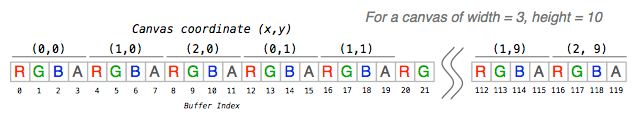# Day 3 – Play with your pixelsThere’s still a metric tonne of turkey left to eat, so let’s work up an appetite 

Canvas provides no obvious way to set an individual pixel, nor read the colour of one… unless you know where to look!

The `getImageData()` function returns an ImageData object, which contains all the raw pixel data. We can choose which part of the canvas that we’re interested in: the (x,y) co-ordinates of the top-left corner, a width and a height.

```// assuming a <canvas> tag with id "canvas" var canvas = document.getElementById("canvas"), context = canvas.getContext("2d"), // we're interested in the full area of the canvas imagedata = context.getImageData(0, 0, canvas.width, canvas.height), // the pixel data buffer buffer = imagedata.data;```

The buffer is an array of numbers, and there are four for every pixel; one for each of the red, green, blue and alpha channels. An alpha of 0 is fully transparent, 255 is fully opaque.

Hopefully this excellent coder art helps to clarify things:So, to calculate the index in the array for a given pixel, we multiply the pixel y-coordinate by the width of the buffer, add the pixel x-coordinate, then multiply the whole lot by four:

`var index = (y * width + x) * 4;`

Knowing this, we can now read the value of a pixel for each of the r, g, b, a bytes:

```// read pixel (100, 53) var index = (53 * imagedata.width + 100) * 4, r = imagedata.data[index], g = imagedata.data[index+1], b = imagedata.data[index+2], a = imagedata.data[index+3];```

Setting the pixel is much the same, but write rather than read:

```// full red imagedata.data[index] = 255; // no green imagedata.data[index+1] = 0; // no blue imagedata.data[index+2] = 0; // fully opaque imagedata.data[index+3] = 255;```

If the array has been modified, we need to ‘write’ it back to the canvas, using the `putImageData()` function:

```// draw the buffer back, at canvas position (0, 0) context.putImageData(imagedata, 0, 0);```

Let’s wrap this up with a more concrete example:

```function setPixel(imagedata, x, y, r, g, b, a) { var i = (y * imagedata.width + x) * 4; imagedata.data[i++] = r; imagedata.data[i++] = g; imagedata.data[i++] = b; imagedata.data[i] = a; }   function getPixel(imagedata, x, y) { var i = (y * imagedata.width + x) * 4;   return {r: imagedata.data[i], g: imagedata.data[i+1], b: imagedata.data[i+2], a: imagedata.data[i+3]}; }   var canvas = document.getElementById("canvas"); canvas.width = 400; canvas.height = 300;   var context = canvas.getContext("2d"); var imagedata = context.getImageData(0, 0, canvas.width, canvas.height);   for( var y = 0 ; y < imagedata.height; y++ ) { for( var x = 0 ; x < imagedata.width; x++ ) { // set the colour randomly setPixel(imagedata, x, y, Math.random() * 255, Math.random() * 255, Math.random() * 255, 255); } }```

While these are intentionally trite code snippets, pixel level access allows us to do a ton of cool stuff, including adding filters to images and manipulating html5 video.

Have fun!

This entry was posted in 12 days of CreativeJS, Canvas (2D), Micro Tutorial by Paul King on Posted on .